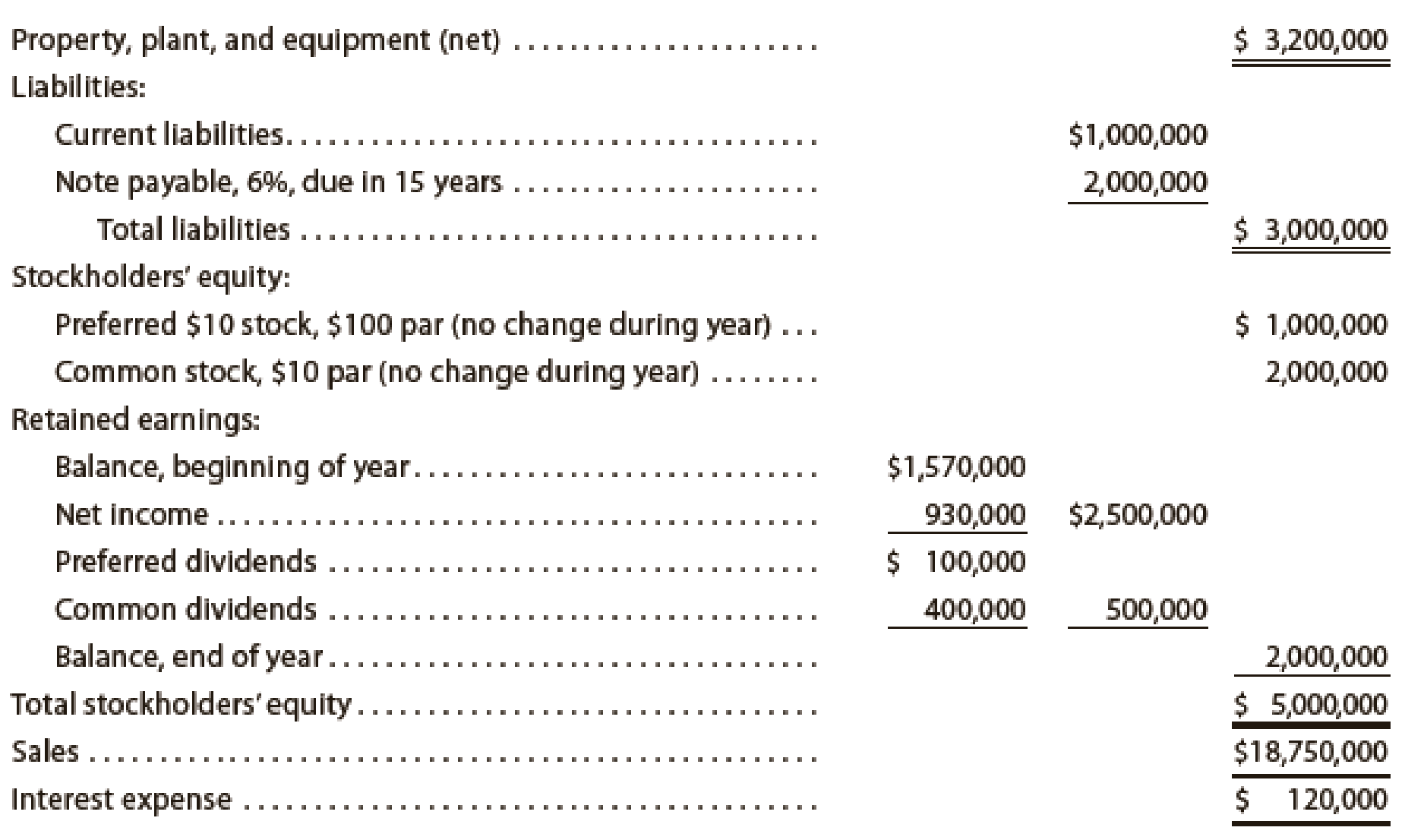Chapter 17, Problem 19E

Chapter
Section
Textbook Problem
1 views

# Six measures of solvency or profitabilityThe following data were taken from the financial statements of Gates Inc. for the current fiscal year.Assuming that total assets were \$7,000,000 at the beginning of the current fiscal year, determine the following: (a) ratio of fixed assets to long-term liabilities, (b) ratio of liabilities to stockholders’ equity, (c) asset turnover, (d) return on total assets, (e) return on stockholders’ equity, and (f) return on common stockholders’ equity. Round ratios and percentages to one decimal place as appropriate.

(a)

To determine

Determine Ratio of Fixed Assets to Long-Term Liabilities.

Explanation

Financial Ratios: Financial ratios are the metrics used to evaluate the liquidity, capabilities, profitability, and overall performance of a company.

Ratio of fixed assets to long-term liabilities is determined by dividing fixed assets and long-term liabilities.

Formula:

Ratio of fixed assets to long-term liabilities=Fixed assets Long-term liabilities

Calculate Ratio of Fixed Assets to Long-Term Liabilities

(b)

To determine

Determine Return on total assets.

(c)

To determine

Determine Asset turnover.

(d)

To determine

Determine return on total assets.

(e)

To determine

Determine return on stockholders’ equity.

(f)

To determine

Determine return on common stockholders’ equity.

### Still sussing out bartleby?

Check out a sample textbook solution.

See a sample solution

#### The Solution to Your Study Problems

Bartleby provides explanations to thousands of textbook problems written by our experts, many with advanced degrees!

Get Started